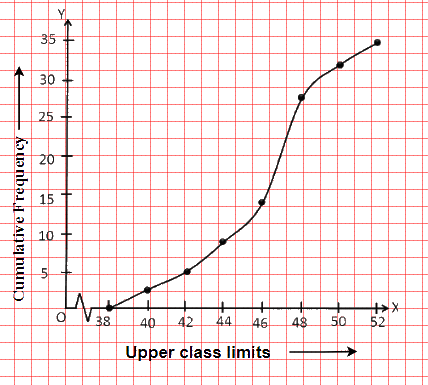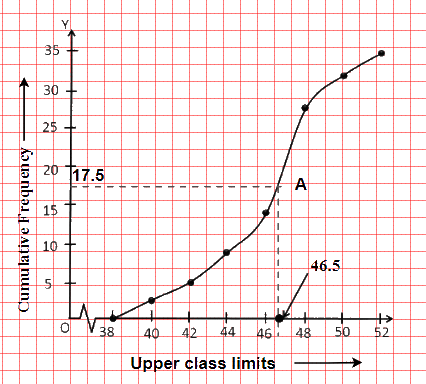# Ex.14.4 Q2 STATISTICS Solution - NCERT Maths Class 10

Go back to  'Ex.14.4'

## Question

During the medical check-up of $$35$$ students of a class, their weights were recorded as follows:

 Weight in (Kg) Number of students Less than $$38$$ $$0$$ Less than $$40$$ $$3$$ Less than $$42$$ $$5$$ Less than $$44$$ $$9$$ Less than $$46$$ $$14$$ Less than $$48$$ $$28$$ Less than $$50$$ $$32$$ Less than $$52$$ $$35$$

Draw a less than type ogive for the given data. Hence obtain the median weight from the graph and verify the result by using the formula.

Video Solution
Statistics
Ex 14.4 | Question 2

## Text Solution

What is known?

The weight of $$35$$ students of a class.

What is unknown?

The less than type ogive and median weight.

Reasoning :

The representation of cumulative frequency distribution graphically is known as a cumulative frequency curve, or an ogive.

Steps:

The given cumulative frequency distributions of less than type are

 Weight in (Kg) Number of students Less than $$38$$ $$0$$ Less than $$40$$ $$3$$ Less than $$42$$ $$5$$ Less than $$44$$ $$9$$ Less than $$46$$ $$14$$ Less than $$48$$ $$28$$ Less than $$50$$ $$32$$ Less than 52 $$35$$

Taking upper class limits on $$x$$-axis and their respective cumulative frequencies on $$y$$-axis, its ogive can be drawn as follows.Here, $$n = 35$$  $$\Rightarrow \frac n{2}=17.5$$

Mark the point $$'A'$$ whose ordinate is $$17.5$$ and its $$x$$-coordinate is $$46.5.$$ Therefore, median of this data is $$46.5.$$It can be observed that the difference between two consecutive upper-class limits is $$2.$$ The class marks with their respective frequencies are obtained as below

 Weight (in Kg) Frequency Cumulative Frequency Less than $$38$$ 0 $$0$$ $$38$$ - $$40$$ $$3 - 0 = 3$$ $$3$$ $$40$$ - $$42$$ $$5 - 3 = 2$$ $$5$$ $$42$$ - $$44$$ $$9 - 5 = 4$$ $$9$$ $$44$$ - $$46$$ $$14 - 9 = 5$$ $$14$$ $$46$$ - $$48$$ $$28 - 14 = 14$$ $$28$$ $$48$$ - $$50$$ $$32 - 28 = 4$$ $$32$$ $$50$$ - $$52$$ $$35 - 32 = 3$$ $$35$$ Total $$n=35$$

Cumulative frequency $$(cf)$$ just greater than $$17.5$$ is $$28$$, beloging to class $$46-48.$$ Therefore,median class $$=46-48$$

Class size ($$h$$) $$= 2$$

Lower class limit of median class ($$l$$)$$=46$$

Frequency of median class ($$f$$)$$=14$$

Cumulative frequency of class preceding median class ($$cf$$) $$=14$$

\begin{align}\text{Median} &= l + \left( {\frac{{\frac{n}{2} - cf}}{f}} \right) \times h\\ &\!=\!46\!+\!\left( \!{\frac{{17.5 - 14}}{{14}}} \!\right)\!\!\times\!\!2\\ &= 46 + \frac{{3.5}}{7}\\ &= 46.5 \end{align}

Therefore, median of this data is $$46.5.$$

Hence, the value of median is verified.

Learn from the best math teachers and top your exams

• Live one on one classroom and doubt clearing
• Practice worksheets in and after class for conceptual clarity
• Personalized curriculum to keep up with school